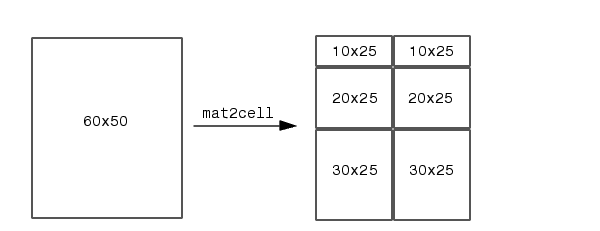MATLAB Function Referencemat2cell

Divide matrix into cell array of matrices

Syntax

• ```c = mat2cell(x, m, n)
c = mat2cell(x, d1, d2, d3, ..., dn)
c = mat2cell(x, r)
```

Description

```c = mat2cell(x, m, n) ``` divides the two-dimensional matrix `x` into adjacent submatrices, each contained in a cell of the returned cell array `c`. Vectors `m` and `n` specify the number of rows and columns, respectively, to be assigned to the submatrices in `c`.

The example shown below divides a 60-by-50 matrix into six smaller matrices. MATLAB returns the new matrices in a 3-by-2 cell array:

• ```mat2cell(x, [10 20 30], [25 25])```

The sum of the element values in `m` must equal the total number of rows in `x`. And the sum of the element values in `n` must equal the number of columns in `x`.

The elements of `m` and `n` determine the size of each cell in `c` by satisfying the following formula for `i` `=` `1:length(m)` and `j` `=` `1:length(n)`:

• ```size(c{i,j}) == [m(i) n(j)]
```

```c = mat2cell(x, d1, d2, d3, ..., dn) ``` divides the multidimensional array `x` and returns a multidimensional cell array of adjacent submatrices of `x`. Each of the vector arguments `d1` through `dn` should sum to the respective dimension sizes of `x` such that, for `p` `=` `1:n`,

• ```size(x,p) == sum(dp)
```

The elements of `d1` through `dn` determine the size of each cell in `c` by satisfying the following formula for `ip` `=` `1:length(dp)`:

• ```size(c{i1,i2,i3,...,in}) == [d1(i1) d2(i2) d3(i3) ... dn(in)]
```

If `x` is an empty array, `mat2cell` returns an empty cell array. This requires that all `dn` inputs that correspond to the zero dimensions of `x` be equal to `[]`.

For example,

• ```a = rand(3,0,4);
c = mat2cell(a, [1 2], [], [2 1 1]);
```

```c = mat2cell(x, r) ``` divides an array `x` by returning a single-column cell array containing full rows of `x`. The sum of the element values in vector `r` must equal the number of rows of `x`.

The elements of `r` determine the size of each cell in `c`, subject to the following formula for `i` `=` `1:length(r)`:

• ```size(c{i},1) == r(i)
```

Remarks

`mat2cell` supports all array types.

Examples

Divide matrix `X` into 2-by-3 and 2-by-2 matrices contained in a cell array:

• ```X = [1 2 3 4 5; 6 7 8 9 10; 11 12 13 14 15; 16 17 18 19 20]
X =
1     2     3     4     5
6     7     8     9    10
11    12    13    14    15
16    17    18    19    20

C = mat2cell(X, [2 2], [3 2])
C =
[2x3 double]    [2x2 double]
[2x3 double]    [2x2 double]

C{1,1}                          C{1,2}
ans =                           ans =
1     2     3                   4     5
6     7     8                   9    10

C{2,1}                          C{2,2}
ans =                           ans =
11    12    13                  14    15
16    17    18                  19    20
```

See Also

`cell2mat`, `num2cell`makehgtform mat2str© 1994-2005 The MathWorks, Inc.